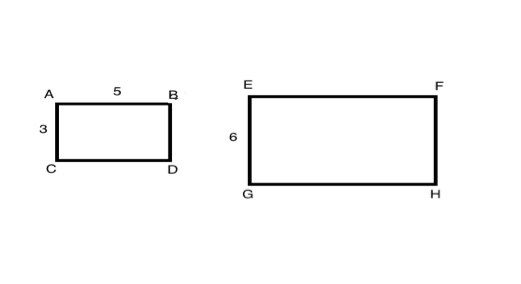# PSAT Math : How to find the length of the side of a rectangle

## Example Questions

### Example Question #621 : Psat Mathematics

The two rectangles shown below are similar. What is the length of EF?10

6

5

8

10

Explanation:

When two polygons are similar, the lengths of their corresponding sides are proportional to each other.  In this diagram, AC and EG are corresponding sides and AB and EF are corresponding sides.

To solve this question, you can therefore write a proportion:

AC/EG = AB/EF ≥ 3/6 = 5/EF

From this proportion, we know that side EF is equal to 10.

### Example Question #11 : Rectangles

A rectangle is x inches long and 3x inches wide.  If the area of the rectangle is 108, what is the value of x?

12

3

6

8

4

6

Explanation:

Solve for x

Area of a rectangle A = lw = x(3x) = 3x2 = 108

x2 = 36

x = 6

### Example Question #31 : Quadrilaterals

If the area of rectangle is 52 meters squared and the perimeter of the same rectangle is 34 meters. What is the length of the larger side of the rectangle if the sides are integers?

12 meters

13 meters

15 meters

16 meters

14 meters

13 meters

Explanation:

Area of a rectangle is = lw

Perimeter = 2(l+w)

We are given 34 = 2(l+w) or 17 = (l+w)

possible combinations of l + w

are 1+16, 2+15, 3+14, 4+13... ect

We are also given the area of the rectangle is 52 meters squared.

Do any of the above combinations when multiplied together= 52 meters squared? yes 4x13 = 52

Therefore the longest side of the rectangle is 13 meters